# Rules of Thumb: Time to Drain a Tank

Article by Stephen Hall

Stephen Hall discusses the golden rules for design

FLUID flow principles can be used to calculate the time needed to drain the contents from a tank. This month I will show you how. The formulas for tanks with simple geometries can be integrated to give an elegant solution. You can approximate the time it takes to drain a tank for other shapes by using the integrated form from a similar tank, or by performing stepwise calculations to integrate the formulas using brute force, the method that I am describing here.

We encounter the tank-draining problem in different forms. How long will it take for a tank to empty if the bottom valve is left open? What is the flow rate from a head tank that flows by gravity into a process vessel? What air pressure is needed to push X liters/minute from tank A to tank B? The solution for each of these problems follows the same form:

1. Balance the driving force, which is the liquid head, against the pressure drop due to friction, and
2. Calculate the change in level over time (the level differential).

As the level in the tank decreases, so does the driving force, which reduces the flow rate. You can set up differential equations to describe this dynamic behaviour. I emphasise you because I am a little shy with applying the mathematics, but explicit solutions are too complex for all but the simplest tank geometries so the brute force approach is often the only option. The computer makes this easy.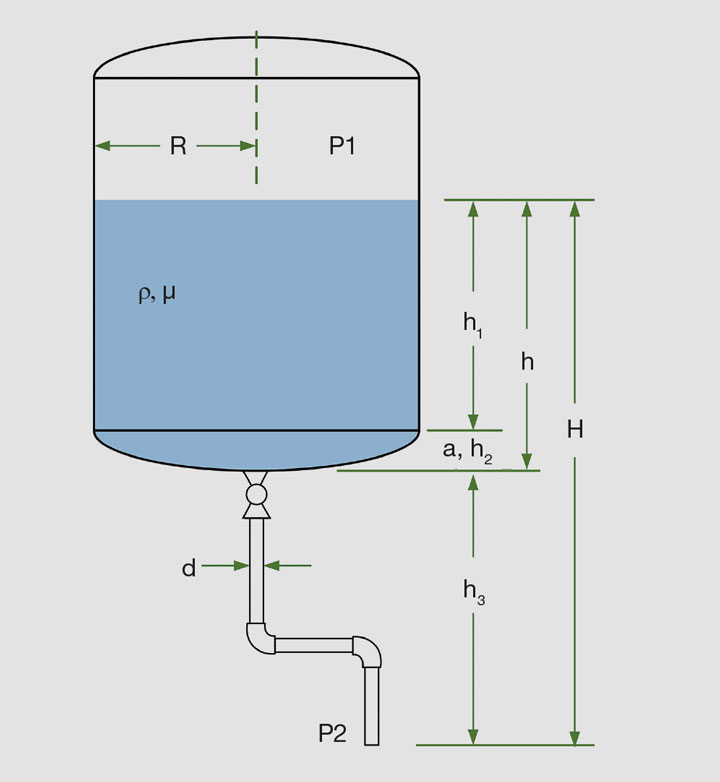Figure 1: Tank nomenclature

Start by sketching the system. The sketch will clearly illustrate the tank with dimensions, head pressure, discharge piping (if any), and discharge pressure. Add the fluid density and viscosity to the sketch (see Figure 1). Using the equations in Table 1, calculate the initial discharge flow rate from this information. Choose a time interval for the calculation, such as 5 or 10 minutes. Assuming the flow rate is constant over the time interval, calculate the volume that flows from the tank and subtract it from the inventory in the tank. From this new inventory calculate the reduced level in the tank (see Table 2 for formulas). This changes the liquid head for the flow rate calculation so recalculate the flow and repeat until the tank is empty.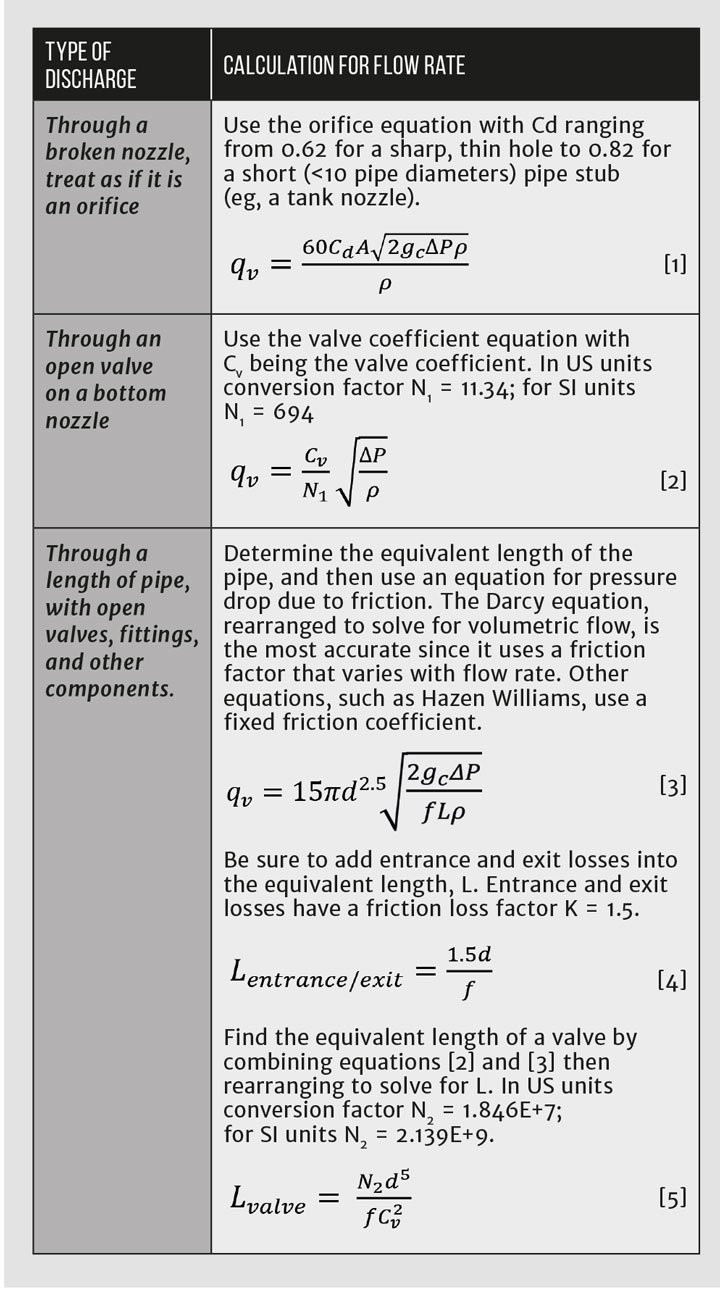Table 1: Flow rate formulas for different discharge scenarios from a tank. In each case the pressure drop accounts for the terminal pressures and the liquid head. The resulting units are volumetric m3/min or ft3/min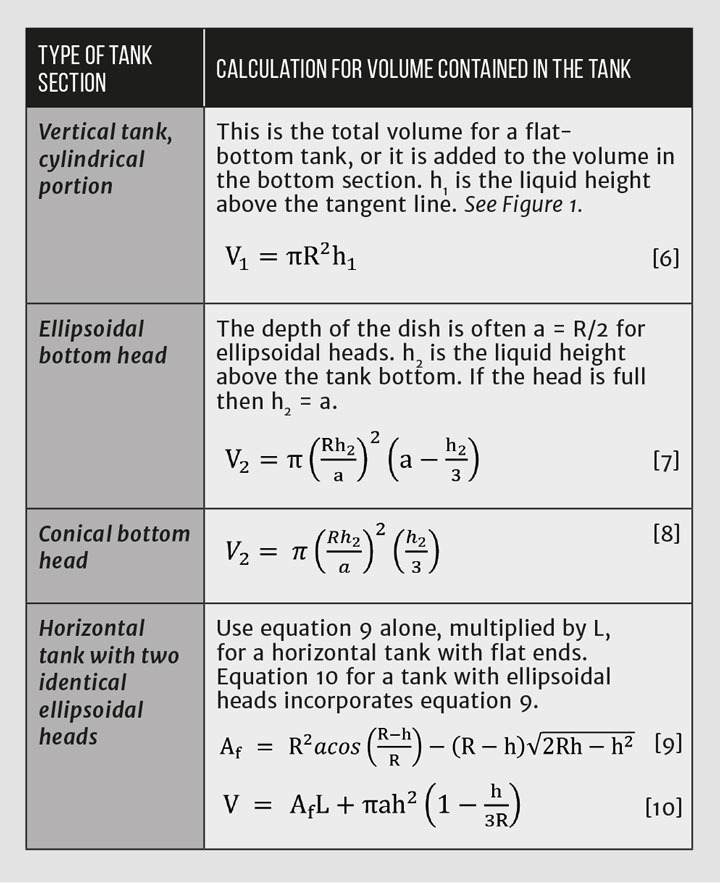Table 2: Volume contained in a tank at a specific fill height

Review the calculation to confirm that the time interval gives a meaningful, but not excessive, change in flow rate. If the interval is too short, there will be many unnecessary calculations. If it is too long, the total estimated time will be low because the flow rate will decrease continuously as the tank level goes down.

The calculation will under-predict the draining time if a vortex reaches into the outlet pipe. A vortex may form when the level gets near the outlet from the tank. Vortex behaviour is complex. It is affected by the velocity of the fluid as it leaves the tank, the tank geometry, and location and orientation of the outlet. The liquid head used for the tank-draining calculations should be the level of the bulk fluid in the tank; a vortex does not change it. If the cone of the vortex reaches into the outlet pipe, the effective flow area of the outlet is decreased, which then decreases the flow rate out of the tank.

The Darcy friction factor that is used in pressure drop equations is determined from the Reynolds number. Without knowing the flow rate, the Reynolds number cannot be calculated. You can handle this problem in one of three ways:

1. Pick a friction factor based upon a turbulent Reynolds number, say 20,000, and keep it constant throughout the analysis;
2. Iteratively calculate the flow rate using Excel’s Goal Seek function or by allowing circular references in the spreadsheet; or
3. For each step in a stepwise calculation, use the flowrate from the previous step to calculate the friction factor for the current step.

The first approach is acceptable, especially for low viscosity fluids that will remain in the turbulent flow range throughout the tank-draining process, but the third method is more satisfying because it removes doubt about the choice of friction factor.

Figures 2–4 and Table 3 present the complete solution for implementation with Excel.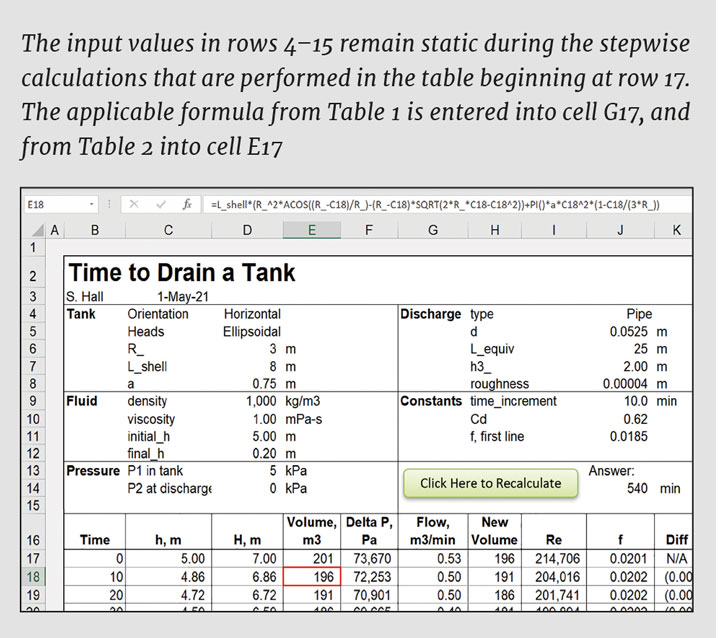Figure 2: Layout of a stepwise tank-draining calculation in a spreadsheet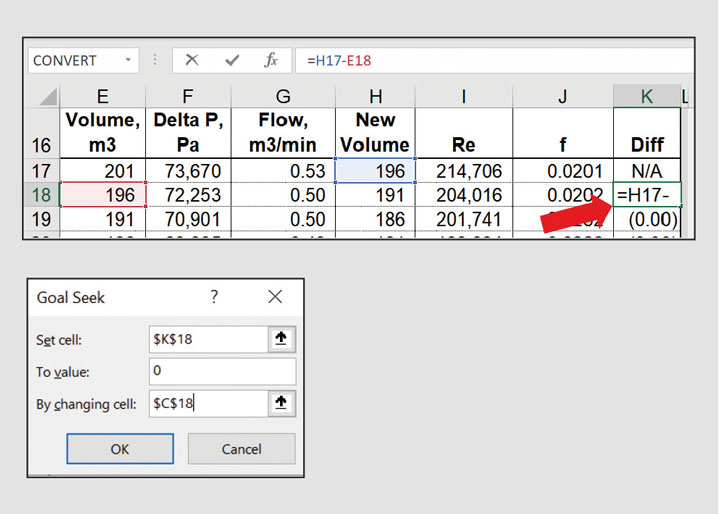Figure 3: Add (Flow*Time_Increment) to Volume to get the New Volume. Guess a new h in Column C, using it to calculate the corresponding volume in Column E. Use Excel’s Goal Seek function to determine h

Sub TankDrain()

'

' Calculates the level in a tank

' by using Excel's Goal Seek function

'

Set h = Range("H")

Set Diff = Range("Diff")

target = Range("Final_h").Value

On Error Resume Next

i = 1

Do

i = i + 1

h.Offset(i) = h.Offset(i - 1)       ' Needed to ensure convergence

Diff.Offset(i).GoalSeek Goal:=0, ChangingCell:=h.Offset(i)

If h.Offset(i) <= target Or Range("New_Volume").Offset(i) < 0 Then

Exit Do

End If

Loop While i < 100 And h.Offset(i + 1, -1) > 0

End Sub

Figure 4: Automate the calculations with Visual Basic for Applications. Add the subroutine shown here and link a Rectangle Shape to it. Click the Shape and the VBA subroutine will execute Goal Seek for each line in the table or until the “final_h” value is reachedTable 3: Formulas in Row 17–18. After entering the formulas in Row 18, copy them down for 20 or more rows. Name the Row 16 headings “Time” (B16), “h” (C16), “New_Volume” (H16), and “Diff” (K16)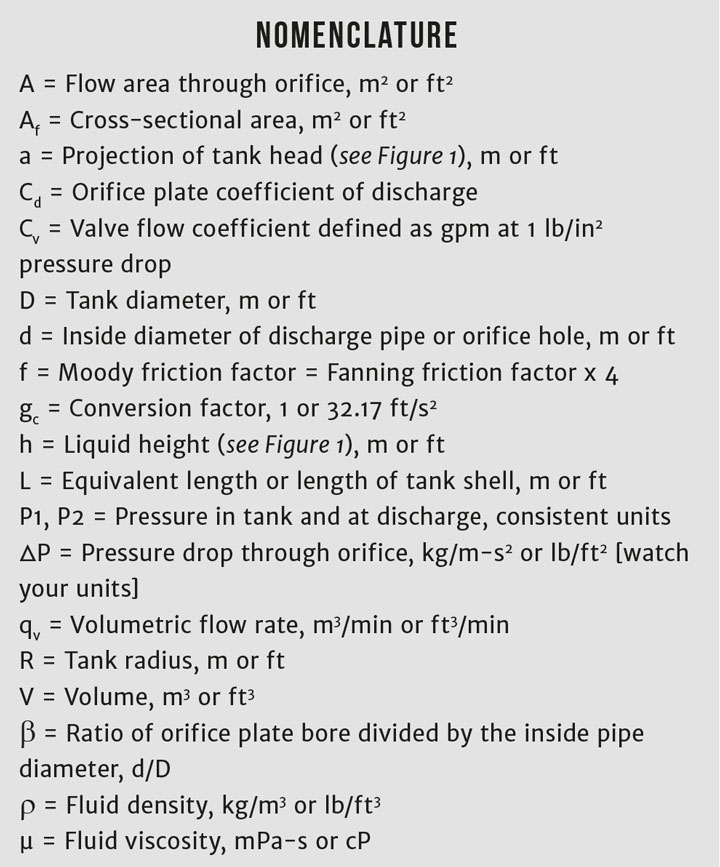This is the ninth in a series that provides practical insights into on-the-job problems. To read more, visit the series hub at https://www.thechemicalengineer.com/tags/rules-of-thumb.